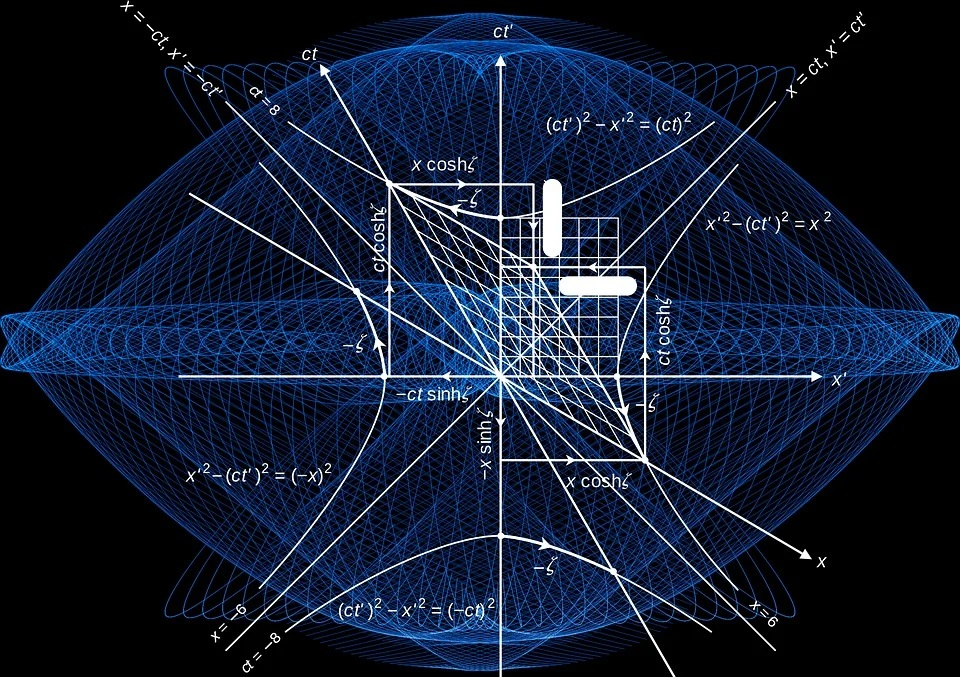## Coordinate Geometry (Analytical Geometry) Class 10th

Introduction Coordinate geometry is the topic in which we shall study coordinates of a point, coordinate axes, Cartesian system, plotting of a point, etc. We use coordinates in analytical geometry so it is called Coordinate Geometry.   Coordinates in Cartesian Plane In the Cartesian plane, there are two number lines that are perpendicular to each …